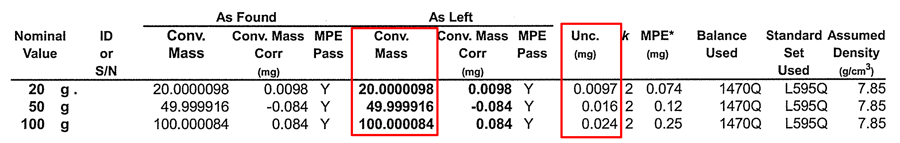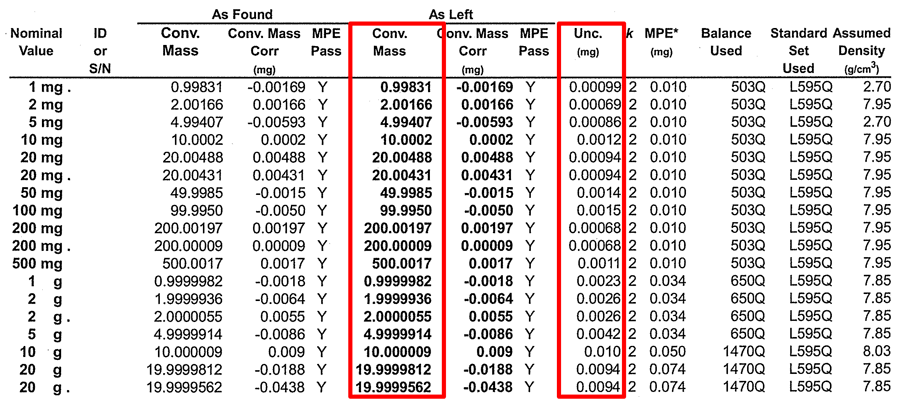# Absolute Uncertainty – Everything You Need to Know## Introduction

Absolute uncertainty and relative uncertainty are two terms used in uncertainty analysis to express how a reported uncertainty should be interpreted. The distinction between the two terms causes many people to be confused.

So, I decided to make this guide to help answer common questions that people ask. Click any of the links below to jump ahead to a particular section.

An absolute uncertainty is expressed in the same unit of measurement as its associated result.

A relative uncertainty is expressed in a term relative to its associated measurement result.

## What is Absolute Uncertainty?

Absolute uncertainty is measurement uncertainty expressed in the same unit of measurement as the measured quantity value.

It is not defined in the Vocabulary in Metrology (VIM), but relative uncertainty is defined in the document. The definition states that the uncertainty is relative to the absolute value. Therefore, we can conclude that absolute uncertainty is associated with the absolute value and expressed in the same units of measurement.

In the image below, you will see an example of a measurement result with its associated absolute uncertainty.Expressing uncertainty as an absolute value is the most common form of reporting uncertainty. Most test and calibration reports report measurement uncertainty as an absolute uncertainty.

## Absolute Uncertainty Example

For example, the certificate below reports the results of a mass calibration where the results are expressed in grams (g) and the expanded uncertainty is expressed in milli-grams (mg). Since the measurement uncertainty and the measured value are both expressed in the same unit of measurement, the reported uncertainty is an absolute uncertainty.This is different from relative uncertainty where the measurement uncertainty is expressed in term relative to the measured quantity value such as percentage or parts in 106.

## How to Calculate Absolute Uncertainty

To calculate absolute uncertainty, you need to perform uncertainty analysis. The most recommended guide for uncertainty analysis is the JCGM 100:2008 – Guide to the Expression of Uncertainty in Measurement which is commonly referred to as the “GUM.”

To perform uncertainty analysis, follow the 7 Steps to Calculate Uncertainty listed below:

1. Specify the Measurement Function,
2. Identify the Sources of Uncertainty,
3. Quantify the Sources of Uncertainty,
4. Characterize the Sources of Uncertainty,
5. Convert the Uncertainties to Standard Uncertainty,
6. Calculate the Combined Uncertainty, and
7. Calculate the Expanded Uncertainty.

When performing uncertainty analysis, make sure the estimated uncertainty is expressed in the same unit of measurement as the evaluated measurement quantity. This will ensure that you calculate the absolute uncertainty.

For example, if you are estimating uncertainty for mass measurements in milligrams, make sure the uncertainty is expressed in milligrams.If you estimated uncertainty using relative uncertainty, you can convert the result to absolute uncertainty. To convert to absolute uncertainty, refer to the section later in this guide.

## How to Find Absolute Uncertainty?

Finding the absolute uncertainty of a measurement quantity can be accomplished in several ways. You can find it by one or more of the following methods:

1. Perform uncertainty analysis,
2. Review an accredited laboratory’s scope of accreditation,
3. Review manufacturer’s specifications, application notes, or white papers,
4. Conference papers or journal articles.

## Difference Between Absolute vs Relative Uncertainty

Both absolute and relative uncertainty express the measurement uncertainty associated with a measurement value. However, the main difference between them is how they are expressed or reported.

Here is a quick summary of their differences:

• Absolute uncertainty is expressed in the same unit of measurement, and
• Relative uncertainty is expressed in a term relative to the measurement value.

## Convert Relative Uncertainty to Absolute Uncertainty

Sometimes you need to calculate absolute uncertainty and only have the relative uncertainty in percent or parts-per-million. Relative uncertainty can be easily converted to an absolute uncertainty using the formulas and instructions below. All you need to know is the measured value and the relative uncertainty.

### Calculate Absolute Uncertainty from Relative Uncertainty (Percentage)

To convert relative uncertainty in percentage to absolute uncertainty, use the formula and instructions provided below.Where,
Urel = Relative Uncertainty (%)
Uabs = Absolute Uncertainty
MVi = Measured Value

Follow the instructions below to calculate absolute uncertainty from percent uncertainty.

1. Multiply the measured value (MV) and the absolute uncertainty (Urel).
2. Divide the result of step 1 by 100.### Calculate Absolute Uncertainty from Relative Uncertainty (Parts per Million)

To convert relative uncertainty in percentage to absolute uncertainty, use the formula and instructions provided below.Where,
Urel = Relative Uncertainty (ppm)
Uabs = Absolute Uncertainty
MVi = Measured Value

Follow the instructions below to calculate absolute uncertainty from ppm uncertainty.

1. Multiply the measured value (MV) and the absolute uncertainty (Urel).
2. Divide the result of step 1 by 1 000 000.## Conclusion

Absolute uncertainty is a common term used in uncertainty analysis and manufacturer specifications. However, it has not been well defined in standards documents and industry guides. As a result, many people have had questions about absolute uncertainty.

In this guide, you should have found answers to common questions about absolute uncertainty, including:

1. What is absolute uncertainty?
2. How to calculate absolute uncertainty?
3. How to find absolute uncertainty?
4. What is the difference between absolute and relative uncertainty?
5. How to convert relative uncertainty to absolute uncertainty?